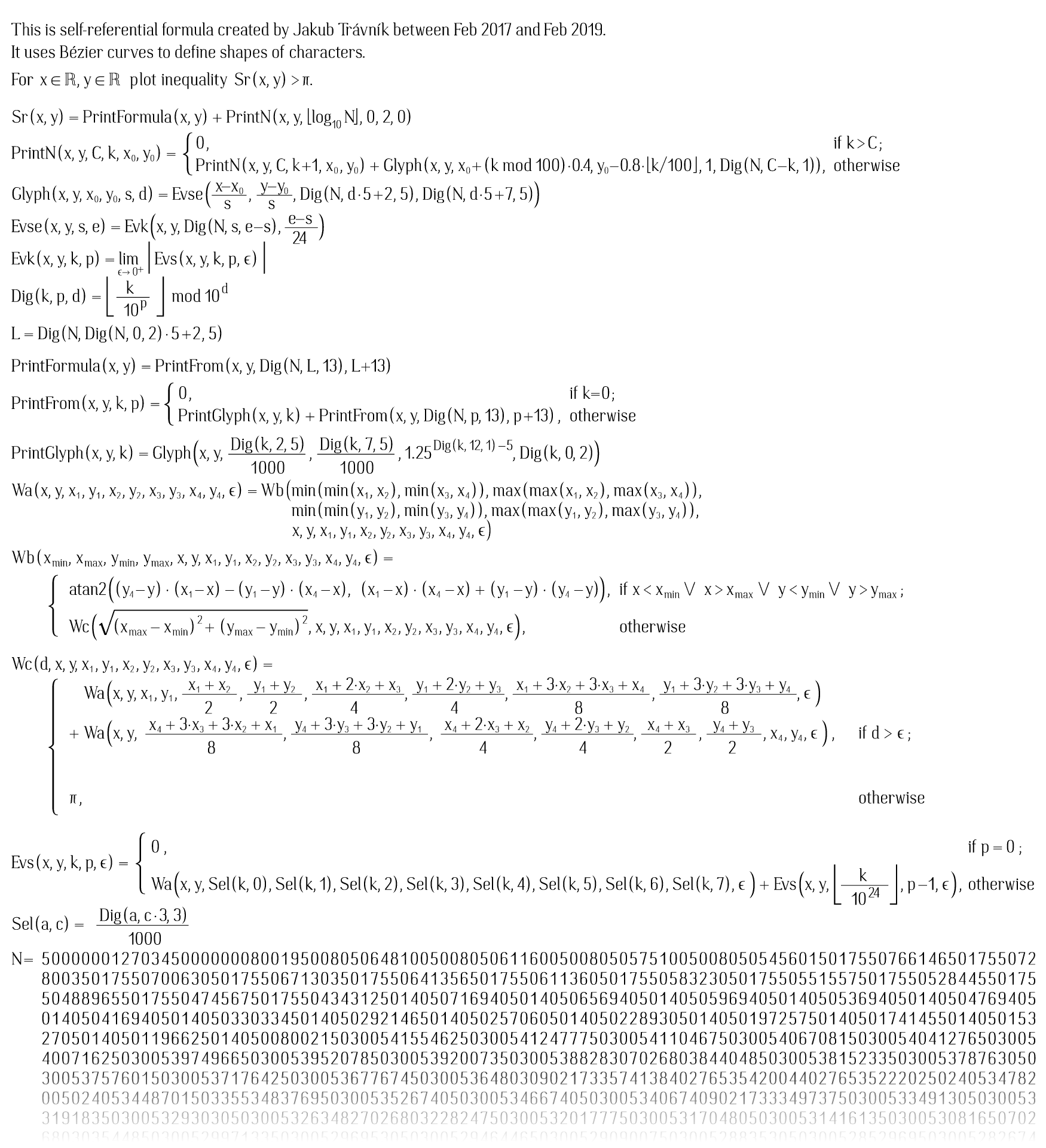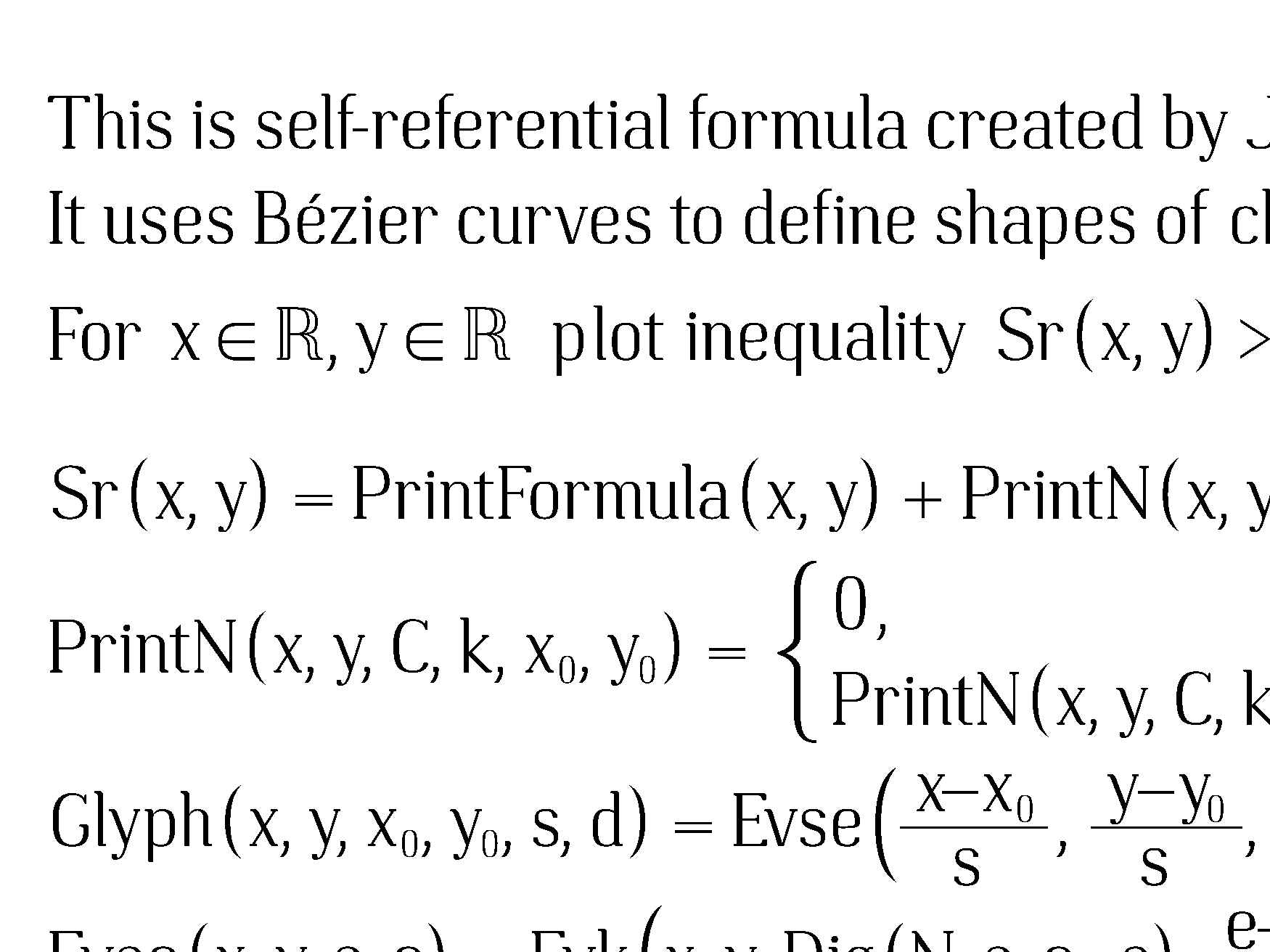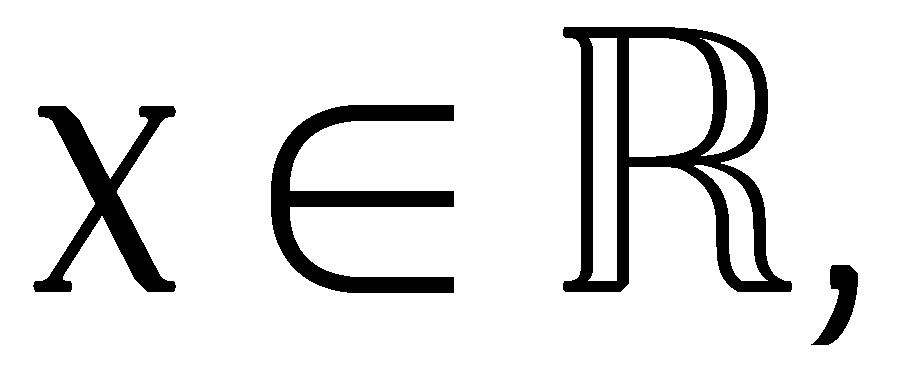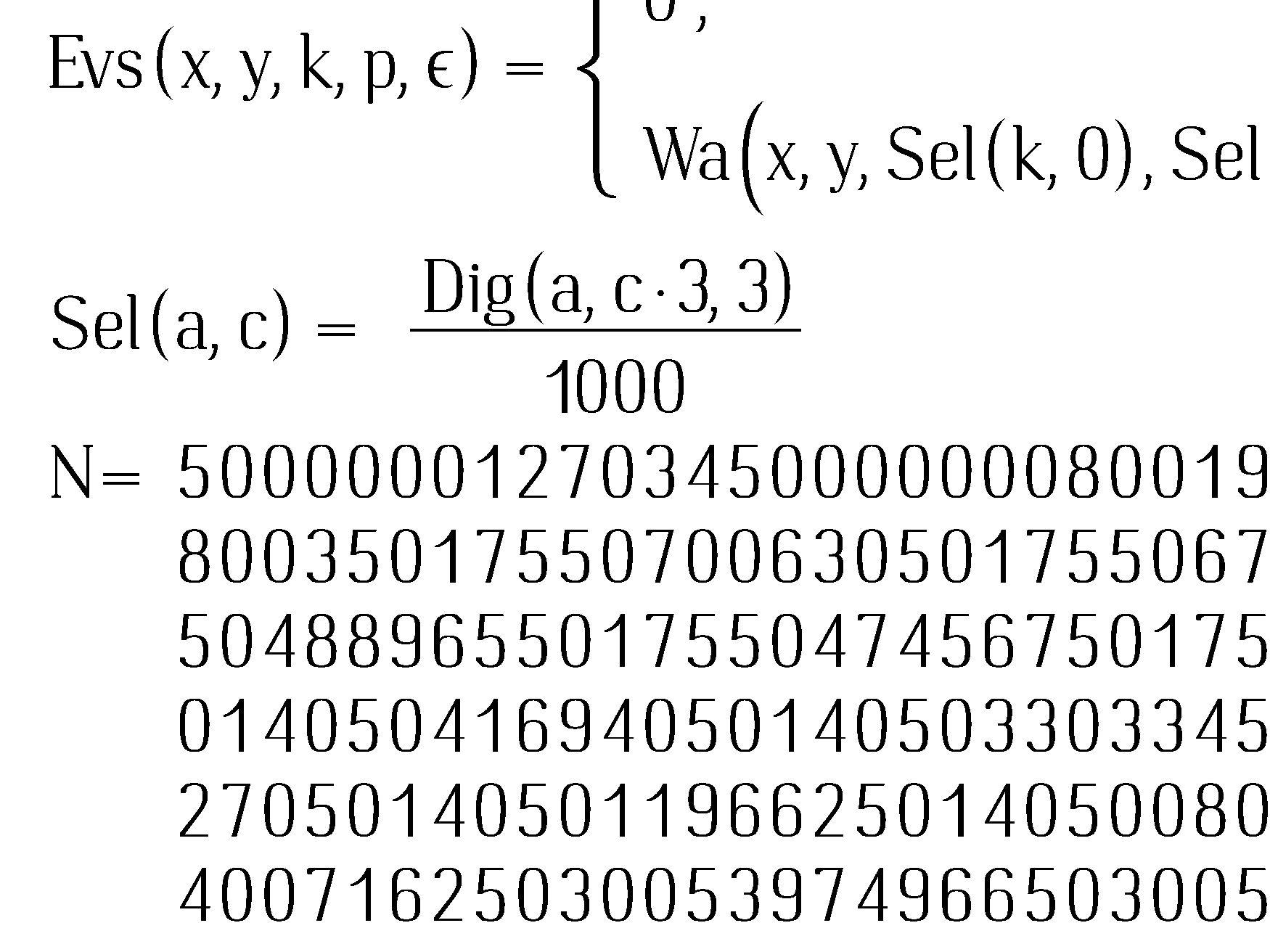# Trávník's smooth self-referential formula

Published: 17 Feb 2019
Updates: see list of updates at the bottom.
Keywords: math, self-reference, quine, smooth, Trávník, Bézier, Tupper

## What is this?

This is a mathematical curiosity. It does not have much practical use. Self-referential formulas have been known for some time, but this one is somewhat special in that it uses Bézier curves.

## Quick history of self-referential formulas

Jeff Tupper is known for what many people call a "self-referential formula".

That one is very simple. Its graph contains all possible bitmaps that fit in region of 17⋅106 grid. So it is not much of wonder that one of those many bitmaps contains meaningful representation of the formula itself. Actually, there multiple ways to fit the formula in 17⋅106 grid, so there are multiple occurrences of the formula in the graph. But there are also all other formulas that fit. It is not truly self-referential since number N which you need to find right viewport is not included inside. Jeff Tupper has produced additional formulas that are truly self-referential, but this famous one is not. You can find them at http://www.peda.com/selfplot/. Timestamps of the files there indicate year 2007.

I have produced a full self-referential formula in 2011. At the time I was only aware of the Tupper's original 2001 formula, but not of his other fully self-referential formulas.

All of the self-referential formulas above were based on encoding bitmaps. Formulas and numbers when plotted using real-valued X and Y axis zoomed would be just composed from squares (usually colored as black and white depending on 1 bit of information) in a grid which is called a bitmap by programmers.

Ever since creating my own self-referential formula in 2011 I have dreamt of creating one that would not be bitmap based. In February 2017 I started with it and completed it in February 2019. Details on its development are available here.

## Here is the formulaThis is the formula, but the N number is quite long. I had to cut off bottom part here. To see it fully, you will find it in linked picture below. It is really big picture with 1750x18625 pixels (33 megapixels), mostly because the N number is really long, it has 50930 decimal digits. Image is so big that it may crash browser/operating system on older smartphones and older computers with low memory.
Click to see formula with complete N. Warning: very big resolution, 2.5MB.
You can also get N in text file here.

This complete view corresponds to x from 0.5 to 42.5 and y from -408 to 39 (the view above with bottom cut off was cut off at around y=-7).

This rendering of formula is the rendering that the formula produced itself. Of course, the formula as displayed requires mathematician to interpret it and it would be tedious to do it by hand. Instead, the rendering is produced by a program (source is available below). I have created two program variants, one where the formula is 1:1 with the formula in as displayed and other which is more optimized but it still very much corresponds to formula as it displays itself. The rendering above was made by optimized formula because of significant computing time requirement to compute that many pixels. Optimizations are described in section on Program Sources and Optimization. You can also try program that plots formula with unoptimized formula, but practically it will work only for very small pictures in reasonable run time.

For viewing pleasure, the image above comes from 8 slightly shifted renderings averaged to produce antialiased image (while increasing run time 8 times).

The formula is based on Bézier curves defining shapes of letters, numbers and other symbols in it. So it can be zoomed without any pixelation. You may have noticed the limit inside the formula. Don't worry, while smaller epsilon values will produce shapes more accurately following Bézier curves, it is sufficient to pick small enough number and go with it. For example all renderings here are with epsilon = 0.00001. See The limit and role of epsilon section. More zoomed renderings follow, this time without antialiasing:

Zoomed rendering: Top left corner:Even more zoomed area from above zoomed image, around x ∈ R:Zoomed area around "N =" part: